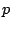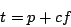Next: Exercises Up: Examples Previous: Overlapping sample looper   Contents   Index

Example B11.sampler.rockafella.pd, shown in part (b) of Figure 2.16, adapts the ideas shown above to a situation where the read point is computed automatically. Here we precess the read-point through the sample in a loop, permitting us to speed up or slow down the playback independently of the transposition.

This example addresses a weakness of the preceding one, which is that, if the relative precession speed is anywhere near one (i.e., the natural speed of listening to the recorded wavetable), and if there is not much transposition either, it becomes preferable to use larger grains and lower the frequency of repetition accordingly (keeping the product constant to achieve the desired transposition.) However, if the grain size is allowed to get large, it is no longer convenient to quantize control changes at phase wrappings, because they might be too far apart to allow for a reasonable response time to control changes.

In this patch we remove the samphold~ object that had controlled the read point (but we leave in the one for chunk size which is much harder to change in mid-loop). Instead, we use the (known) rate of precession of the read point to correct the sawtooth frequency, so that we maintain the desired transposition. It turns out that, when transposition factor and precession are close to each other (so that we are nearly doing the same thing as simple speed change) the frequency will drop to a value close to zero, so we will have increased the naturalness of the result at the same time.

In this patch we switch from managing read points, chunk sizes, etc., in samples and use seconds instead, converting to samples (and shifting by one) only just before the tabread4~ object. The wavetable holds one second of sound, and we'll assume here that the nominal chunk size will not exceed 0.1 second, so that we can safely let the read point range from 0 to 0.9; the real" chunk size will vary, and can become quite large, because of the moving read pointer.

The precession control sets the frequency of a phasor of amplitude 0.9, and therefore the precession must be multiplied by 0.9 to set the frequency of the phasor (so that, for a precession of one for instance, the amplitude and frequency of the read point are both 0.9, so that the slope, equal to amplitude over frequency, is one). The output of this is named read-pt" as before, and is used by both copies of the wavetable reader.

The precessionand the chunk sizebeing known, and if we denote the frequency of the upper (original) phasor~ by, the transposition factor is given by:and solving forgives:whereis the desired transposition in half-steps. This is the formula used in the expr object.Next: Exercises Up: Examples Previous: Overlapping sample looper   Contents   Index
Miller Puckette 2006-12-30